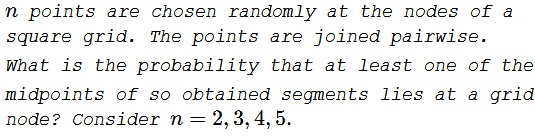Points on a Square Grid

ProblemSolution 1

Given two grid points, their $x-\text{coordinates}$ have the same parity with the probability of $\displaystyle \frac{1}{2}.$ Same for the $y-\text{coordinates}.$ Both coordinates have (pairwise) the same parity with the probability of $\displaystyle \frac{1}{2}\cdot\frac{1}{2}=\frac{4}{2}.$

For $n=3,$ for any combination of the coordinates of one of them there are six combinations of parities for the other two that do not lead to a midpoint at a grid node. For example, if one point has both coordinates even, denoted $e/e,$ then for the other two there are the following possibilities:

$\begin{cases} e/o,&o/e,o/o\\ o/e,&o/o,o/e\\ o/o,&o/e,e/o \end{cases}$

Since there are four combinations of parities for the chosen point, there are $4\cdot 6=24$ possible combinations that do not place a midpoint at a grid node. For three points, there are $\displaystyle 4^3$ of possible combinations so that the probability of getting a midpoint on a grid node equals $\displaystyle 1-\frac{24}{64}=\frac{5}{8}.$

For, $n=4,$ there is no midpoint on a grid, if and only if, the four points have all four distinct parity combinations: $o/o,$ $o/e,$ $e/o,$ $e/e.$ There are $4!$ ways to distribute these combinations between the four points, out of the total of $4^4=256$ combinations. This happens with the probability $\displaystyle \frac{24}{256}=\frac{3}{32}.$ Thus, at least one of the midpoints falls on a grid node with the probability of $\displaystyle 1-\frac{3}{32}=\frac{29}{32}.$

For $n=5,$ it is shown elsewhere with the Pigeonhole principle that in this case at least one of the midpoints falls onto a grid node. On the other hand, since there are only four possible parity combinations for a 2D grid point, if there are five points, the probability is $1$ that two of them have the same combination of parities. This may be considered a probabilistic solution to an otherwise deterministic problem.

Solution 2

Each point has four equally likely parity combinations (odd/even on both coordinates). No midpoint will be a grid node when no two points have the same parity, for which the probability is

$\displaystyle\begin{cases} n=2,& \displaystyle \left(\frac{1}{4}\right)^2\cdot 4\cdot 3 = \frac{3}{4}\\ n=3,& \displaystyle \left(\frac{1}{4}\right)^3\cdot 4\cdot 3\cdot 2=\frac{3}{8}\\ n=4,& \displaystyle \left(\frac{1}{4}\right)^4\cdot 4\cdot 3\cdot 2\cdot 1=\frac{3}{32}\\ n=5,& \displaystyle \left(\frac{1}{4}\right)^5\cdot 4\cdot 3\cdot 2\cdot 1\cdot 0=0\;\text{ (unauthorized addition)} \end{cases}$

The probability that a midpoint will fall at the node of the grid is $\displaystyle 1-\frac{3}{4}=\frac{1}{4},$ $\displaystyle 1-\frac{3}{8}=\frac{5}{8},$ $\displaystyle 1-\frac{3}{32}=\frac{5}{32},$ $1=1-0,$ for $n=2,3,4,5,$ respectively.

Solution 3

The nodes can be considered to have integral $X$ and $Y$ coordinates. For midpoints to have integral coordinates (with probability denoted by $P),$ the coordinates of both points should have same parity - even or odd (a requirement for both $X$ and $Y$ coordinates). For each point, there are four possibilities (four bins) with equal probability: $(e,e)$, $(e,o)$, $(o,e)$, and $(o,o)$ (brackets show the parity for the $X$ and $Y$ coordinates).

For $n\gt 4$, pigeonhole principle guarantees that there will be at least two points in the same bin and $P(n:n>4)=1$.

For the remaining cases, $P$ is one minus the probability of assigning distinct bins (Assign first point to a random bin, the second point can be assigned to one of the remaining three bins, third point to one of the remaining two bins and so on). Thus,

$\displaystyle P(n)= \begin{cases} \displaystyle 1-\prod_{k=1}^{n-1}\frac{(4-k)}{4} & (n\leq 4), \\ 1 & (n\gt 4). \end{cases}$

Hence,

\displaystyle \begin{align} P(2)&=1-\frac{3}{4}=\frac{1}{4},\\ P(3)&=1-\frac{3}{4}\cdot\frac{2}{4}=\frac{5}{8},\\ P(4)&=1-\frac{3}{4}\cdot\frac{2}{4}\cdot\frac{1}{4}=\frac{29}{32},\\ P(5)&=1. \end{align}

Acknowledgment

The problem came up as a variant of an earlier exercise in the Pigeonhole principle. Solution 2 is by twitter user umtab @dash_amitabh; Solution 3 is by Amit Itagi.

Geometric Probability